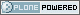# Neuroimaging Informatics Technology Initiative

##### Personal tools
You are here: Home » xyzt_units.html

# xyzt_units.html

## xyzt_units

```
/---------------------------------------------------------------------------/
/* UNITS OF SPATIAL AND TEMPORAL DIMENSIONS:
----------------------------------------
The codes below can be used in xyzt_units to indicate the units of pixdim.
As noted earlier, dimensions 1,2,3 are for x,y,z; dimension 4 is for
time (t).
- If dim=1 or dim < 4, there is no time axis.
- A single time series (no space) would be specified with
- dim = 4 (for scalar data) or dim = 5 (for vector data)
- dim = dim = dim = 1
- dim = number of time points
- pixdim = time step
- xyzt_units indicates units of pixdim
- dim = number of values stored at each time point   Bits 0..2 of xyzt_units specify the units of pixdim[1..3]
(e.g., spatial units are values 1..7).
Bits 3..5 of xyzt_units specify the units of pixdim
(e.g., temporal units are multiples of 8).
This compression of 2 distinct concepts into 1 byte is due to the
limited space available in the 348 byte ANALYZE 7.5 header.  The
macros XYZT_TO_SPACE and XYZT_TO_TIME can be used to mask off the
undesired bits from the xyzt_units fields, leaving "pure" space
and time codes.  Inversely, the macro SPACE_TIME_TO_XYZT can be
used to assemble a space code (0,1,2,...,7) with a time code
(0,8,16,32,...,56) into the combined value for xyzt_units.
Note that codes are provided to indicate the "time" axis units are
actually frequency in Hertz (_HZ), in part-per-million (_PPM)
The toffset field can be used to indicate a nonzero start point for
the time axis.  That is, time point #m is at t=toffset+m*pixdim
for m=0..dim-1.
```

### DEFINED CODES

```#define NIFTI_UNITS_UNKNOWN 0 /! NIFTI code for unspecified units. /                               / Space codes are multiples of 1. /
#define NIFTI_UNITS_METER   1 /! NIFTI code for meters. /
#define NIFTI_UNITS_MM      2 /! NIFTI code for millimeters. /
#define NIFTI_UNITS_MICRON  3 /! NIFTI code for micrometers. /
/ Time codes are multiples of 8. /
#define NIFTI_UNITS_SEC     8 /! NIFTI code for seconds. /
#define NIFTI_UNITS_MSEC   16 /! NIFTI code for milliseconds. /
#define NIFTI_UNITS_USEC   24 /! NIFTI code for microseconds. /
/* These units are for spectral data: /
#define NIFTI_UNITS_HZ     32 /! NIFTI code for Hertz. /
#define NIFTI_UNITS_PPM    40 /! NIFTI code for ppm. /
```

### MISCELLANEOUS C MACROS

```#define XYZT_TO_SPACE(xyzt)       ( (xyzt) & 0x07 )
#define XYZT_TO_TIME(xyzt)        ( (xyzt) & 0x38 )
#define SPACE_TIME_TO_XYZT(ss,tt) (  (((char)(ss)) & 0x07)   \
| (((char)(tt)) & 0x38) )
```

Created by Alex Clark